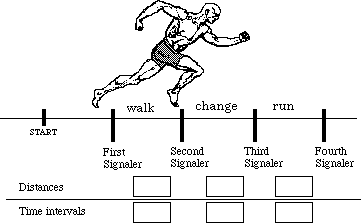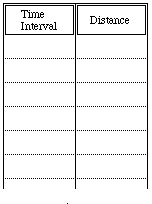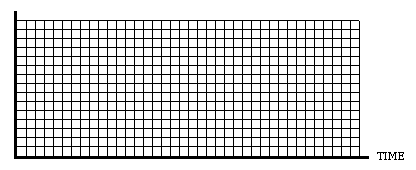# Hands-On-Physics MECHANICS

### Running

Measure multiple time intervals using "lap" timer to analyze the motion of a person who first walks and then speeds up to a run.

Suggestions:
Choose a timer who will measure the time intervals of the runner. Set up a course for the runner and position four signalers along the course to signal the timer when the runner passes their location. One person at the beginning of constant walk, one at the beginning of changing speed, one at the beginning of constant run, and one at the end of constant run. Agree on a signals for the person keeping time. Be sure to measure the distance from start to the first signaler, and distances between signalers. You could, instead, call start zero and measure the positions of the timers with respect to zero.Figure M1
Runner changing speed

Make tables for recording your measurements, an example is shown below. Tables are also helpful for keeping track of calculated values.Figure M2
Time & distance table

ANALYSIS:
To get a clear picture of the motion, several speed calculations are suggested, and two graphs are recommended. Time is generally plotted horizontally.

• Calculate the runner's constant walking speed.
• Calculate the runner's constant running speed.
• What about speed in the middle part of the journey?
• Plot a point for each time your runner passed a signaler. ( a position vs. time graph.) You may want to make a table of values before you begin plotting points.Figure M3a
Runner's position graph
• Think out what the runner's speed was at each of those times and plot a point on the graph for each of those times. (This is a speed vs. time graph.)Figure M.3 b
Runner's speed graph

Previous Page || Up a Level || Index || Next Page